# dipole

(redirected from Dipoles)
Also found in: Dictionary, Thesaurus, Medical, Acronyms.
Related to Dipoles: dipole moment

## dipole:

see polepole,
in electricity and magnetism, point where electric or magnetic force appears to be concentrated. A single electric charge located at a point is sometimes referred to as an electric monopole.
, in electricity and magnetism.

## Dipole

Any object or system that is oppositely charged at two points or poles, such as a magnet, a polar molecule, or an antenna element. The properties of a dipole are determined by its dipole moment, that is, the product of one of the charges by their separation directed along an axis through the centers of charge. See Dipole moment

An electric dipole consists of two electric charges of equal magnitude but opposite polarity, separated by a short distance (see illustration); or more generally, a localized distribution of positive and negative electricity without net charge whose mean positions of positive and negative charge do not coincide.

Molecular dipoles which exist in the absence of an applied field are called permanent dipoles, while those produced by the action of a field are called induced dipoles. See Polar molecule

The term magnetic dipole originally referred to the fact that a magnet has two poles and, because of these two poles, experiences a torque in a magnetic field if its axis is not along a magnetic flux line of the field. It is now generalized to include electric circuits which, because of the current, also experience torques in magnetic fields. See Magnet

An electric dipole whose moment oscillates sinusoidally radiates electromagnetic waves and is known as a hertzian dipole; it is of interest in developing the theory of electromagnetic radiation. For practical purposes, a half-wave dipole, consisting of two collinear conducting rods, fed at the center, whose combined length equals half the wavelength of the radiation to be transmitted or received, is often used as an antenna element, either by itself or in an array, or as a feed for a reflector. See Electromagnetic radiation

## dipole

(dÿ -pohl) A simple linear balanced antenna that is fed from its center. It usually consists of two equal-length straight opposing elements, although these may sometimes be folded back on themselves and their far ends joined together in the middle to form a folded dipole. The elements may be of any length but the overall length of the dipole is usually a multiple of half a wavelength of the received (or transmitted) radio wave; the reactive component of the impedance seen at its feed point is then small.

A half-wave dipole has two elements of length one-quarter of a wavelength each and has a radiation resistance of about 70 ohms. A full-wave dipole is twice as long as a half-wave dipole and has a radiation resistance of several thousand ohms. The gain of a half-wave dipole over an isotropic radiator is about two decibels.

## Dipole

There are two types of dipole, electric and magnetic.

Electric dipole. An electric dipole is the aggregate of two opposite point charges that are equal in absolute value and that are separated by a certain distance. The main characteristic of an electric dipole is the dipole moment, which is a vector directed from the negative charge to the positive charge (see Figure 1), whose magnitude is equal to the product of the charge e and the distance l between the two charges: p = eI. The dipole moment determines the electric field of the dipole at a great distance R from the dipole (R » l), as well as the effect of the external electric field on the dipole.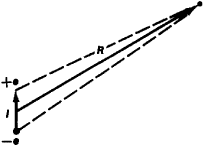Figure 1. An electric dipole: the charges −e and +e are separated by the distance l. The dipole moment of this dipole, p = eI, completely defines the electrical field of the dipole for distances R » l.

In regions remote from the dipole its electric field E decreases with distance as 1/R3, that is, faster than the field of the point charge (~t/R2). The components of field intensity E along the axis of the dipole (Ep) and perpendicular to p (E1) are proportional to the dipole moment. In the cgs (Gaussian) system of units, they are

Ep = (p/R3) (3 cos2 θ − 1) = (3p/R3) (cos θ sin θ)

where θ is the angle between p and the radius vector R of the point in space where the field of the diode is being measured; the total intensity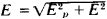. Thus, the field intensity on the axis of the diode for θ = 0 is twice that for θ = 90°. For both of these angles the field intensity has only the component Ep. At θ = 0 the direction of the field intensity is parallel to p; at 0 = 90° it is antiparallel (see Figure 2).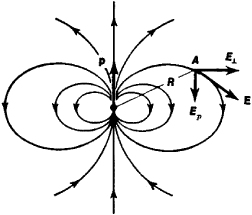Figure 2. Electrical field of a dipole, shown by means of lines of force. At the observation point A, located at a distance R from the center of the dipole (R » l), the field E (directed along a line tangential to the line of force) is resolved into two components, Ep parallel to the axis of the dipole and E. perpendicular to it.

The effect of the external electric field on the dipole is also proportional to the magnitude of its dipole moment. A homogeneous field generates a rotational moment M = pE sin α (α is the angle between the vector of intensity of the external electric field E and the dipole moment p; see Figure 3),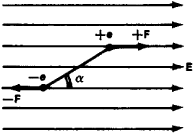Figure 3. A dipole in a homogeneous external electrical field E is acted upon by a pair of forces (−F and +F), which generates a rotational moment that tends to turn the dipole in the direction of the field

which tends to turn the dipole in such a way that its dipole moment is oriented in the direction of the field. In a nonhomogeneous electric field the dipole is acted upon not only by the rotational moment but also by a force tending to pull the dipole into the region of a stronger field (see Figure 4).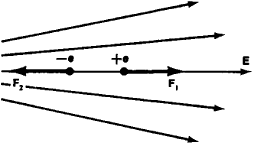Figure 4. An electric dipole in a nonhomogeneous electrical field E in a particular case, when the dipole moment p is directed along the field (greater density of lines of force corresponds to greater intensity of the field). F1 and F2 are forces acting from the field on the charges −e and +e; F2 > F1, and the resultant force F = F2F1, tends to reposition the dipole into a region of greater field intensity.

The electric field of any neutral system approximately coincides with the field of an equivalent dipole if the distance from the system is much greater than its dimensions. An equivalent dipole is one that has the same dipole moment as the charge system in question. (That is, at great distances from the system, the field is insensitive to the details of charge distribution.) Therefore, in many cases an electric dipole is a good approximation for describing such a system at distances much greater than the system’s dimensions. For example, the molecules of many substances may be regarded approximately as electric dipoles (in the simplest case, such molecules consist of two ions with opposite charges); the atoms and molecules in the external field, which separates their positive and negative charges somewhat, acquire a field-induced dipole moment and themselves become microscopic dipoles.

Electric dipoles whose dipole moment changes with time (as a consequence of a change in their length l or charges e) are sources of electromagnetic radiation.

Magnetic dipole. The study of the interactions of the poles of permanent magnets (C. Coulomb, 1785) led to the idea of the existence of magnetic charges analogous to electric charges. A pair of such charges, of equal magnitude but opposite sign, was regarded as a magnetic dipole (having a magnetic dipole moment). Later it was found that magnetic charges do not exist and that magnetic fields are created by moving electric charges—that is, electric currents. However, the preservation of the concept of a magnetic dipole was found to be expedient, since at great distances from a closed conductor loop through which current is flowing, the magnetic fields are identical to the fields that would be produced by a magnetic dipole (at great distances from the dipole, the magnetic field of a magnetic dipole is computed according to the same formulas as are used for the electrical field of an electric dipole, except that the magnetic moment of the current is substituted for the electrical moment of a dipole). The magnetic moment of a current system is defined by the magnitude and distribution of the currents. In the simplest case, with a current I flowing through a loop with radius a, the magnetic moment in the cgs system is p = ISn/c, where S = πa2 is the area of the loop; the unit vector n, drawn from the center of the loop, is directed in such a way that, when viewed from its end, the current is seen as flowing counterclockwise (see Figure 5); and c is the speed of light.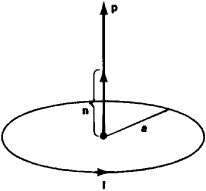Figure 5. Magnetic moment of a circular current

The analogy between a magnetic dipole and a current-carrying conductor loop may also be followed in examining the effects of the magnetic field on a current. In a homogeneous magnetic field the current-carrying conductor loop is subjected to a force moment that tends to rotate the loop in such a way that its magnetic moment assumes the direction of the field; in a nonhomogeneous field such closed-loop currents (“magnetic dipoles”) are pulled into the region of greatest field intensity. This interaction of a magnetic field and a magnetic dipole is the basis of some physical processes, such as the separation of particles with different magnetic moments (nuclei, atoms, or molecules), whose magnetic moments depend on the motion of the charged elementary particles of which they are composed, as well as on the magnetic moments associated with the spin of the particles. A beam of such particles separates upon passing through a nonhomogeneous magnetic field because the change in the trajectory of a particle (caused by the field) is greater for particles with greater magnetic moments.

However, the analogy between a magnetic dipole and a current-carrying conductor loop (the equivalence theory) is not complete. For example, in the center of a conductor loop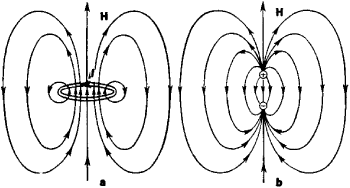Figure 6. Magnetic field near a circular current I (a) and a magnetic dipole (b); at great distances the fields are identical

the magnetic field intensity is not only not equal to the field intensity of an “equivalent” dipole but is also of opposite direction (see Figure 6). The magnetic lines of feree, as distinct from electrical lines offeree, which originate and terminate in charge locations, are closed.

### REFERENCES

Feynman, R., R. Layton, and M. Sands. Feinmanovskie lektsii po fizike, fasc. 5: Elektrichestvo i magnetizm. Moscow, 1966. Chapter 6. [Translated from English.]
Kalashnikov, S. G. Elektrichestvo. Moscow, 1956. Section 17. (Obshchii kurs fiziki, vol. 2.)
Kirenskii, L. V. Magnetizm, 2nd ed. Moscow, 1967.
Tamm, I. E. Osnovy teorii elektrichestva, 7th ed. Moscow, 1957.

G. IA. MIAKISHEV and V. I. GRIGOR’EV

## dipole

[′dī‚pōl]
(electromagnetism)
Any object or system that is oppositely charged at two points, or poles, such as a magnet or a polar molecule; more precisely, the limit as either charge goes to infinity, the separation distance to zero, while the product remains constant. Also known as doublet; electric doublet.

## dipole

1. two electric charges or magnetic poles that have equal magnitudes but opposite signs and are separated by a small distance
2. a molecule in which the centre of positive charge does not coincide with the centre of negative charge
References in periodicals archive ?
First, let us cite in the same sense order, as for the dipole model (see Table 1), the basic equations and magnetostatic formulas for magnetized media based on the model of magnetization by molecular currents [1, 4]:
This design gives the desired magnetic dipole or loop antenna radiation pattern as shown in Figure 8 and Figure 9.
r] results for the three single dipoles cases (radial, axial and tangential) and the three simultaneous dipoles (dilatation) (with source positions on the axis to 90[degrees] line) are best demonstrated in figure 11 using the CWD results for first quadrant signals (at 90 mm) with all the source centers off axis at 1 mm.
where v is the actual propagation speed on the dipole radials and f is the resonant frequency.
Please use the dipole small-magnets model to explain why the iron wires would become magnetic by considering the similarities and differences between the magnet and the iron wires.
The experimental sessions consisted of 4 acclimation bites, in which food was provided at the dipole to which the rays were conditioned, and 10 unrewarded (experimental) bites (see Gerstein, 1994; Gerstein et at.
Kunz, "Light emission by magnetic and electric dipoles close to a plane interface.
21] of diamond dipoles above AMC sheet with varying patch's width.
7 mm was chosen as the length of the horizontal triangular dipole for good impedance matching.
and the radiation fields excited by the magnetic dipole are
Various antenna designs, such as bow-tie , logarithm spiral , strip line , strip line dipole [15,16], and fractal-based antennas [17,18], have received significant attention for better generation and detection of THz radiation .
Furthermore, because the local dipoles do not appear to as readily "rotate" for PMVC (10), the issue of dipole ordering remains poorly addressed.

Site: Follow: Share:
Open / Close